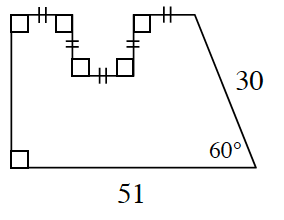### Home > CCG > Chapter 9 > Lesson 9.1.2 > Problem9-20

9-20.Mr. Wallis is designing a home. He found the plan for his dream house on the Internet and printed it out on paper.

1. The design of the home is shown at right. If all measurements are in millimeters, find the area of the diagram.

Divide the shape into smaller, easier shapes.

There is a $30°\text{-}60°\text{-}90°$ triangle. The height is $15\sqrt{3}$ and the base is $15$.

By subtracting the base of the triangle from $51$, you can find the length of the rectangle.

Area $≈ 986.16\text{ mm}^2$

2. Mr. Wallis took his home design to the copier and enlarged it $400\%$. What is the area of the diagram now? Show how you know.

If the design is enlarged by $400\%$, how many times bigger is the shape? How does the area of the new shape change?